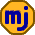## Monday, August 31, 2009

### Studying Financial Markets with Fractals

Benoit Mandelbrot is famous for developing the idea of fractals and showing that many aspects of nature follow fractal-based laws. In his book The (Mis)Behavior of Markets he shows how his theory of fractals applies to financial markets and manages to do it with very little mathematical discussion.

Mandelbrot takes a very visual approach. His starting point is to randomly generate fictitious price charts according to modern portfolio theory and observe that the charts don’t look right. When they are placed next to real price charts, the fakes can be picked out by people just looking at them.

As it turns out, the problem is that extreme price changes are more frequent than portfolio theory predicts, and the bigger price moves tend to come in bunches. The distribution of real price changes doesn’t follow the standard Bell curve.

Mandelbrot makes convincing arguments that price changes follow a distribution that is wilder than a Bell curve. Using fractal-based methods, he is able to generate fictitious price histories that are much more realistic-looking.

Bell curves are used for statistical purposes in many endeavours, and the truth is that it is never exactly the right fit. Any time we model the real world with math the fit isn’t perfect. For example, nothing in the real world is exactly circular. The important question is whether the model is good enough to give useful answers.

Modern portfolio theory is able to give useful answers to some questions, but Mandelbrot shows that it frequently gives wrong answers as well. The main problem is that it underestimates the likelihood of big gains and big losses. A good example of this is David Li’s formula for measuring the risk of default in baskets of mortgages that grossly underestimated the chances of widespread defaults that lead to the recent global financial collapse.

Mandelbrot doesn’t leave us with any single solution to the problem of figuring out what we should own in our portfolios. One thing for certain is that we need to be prepared in the future for the kind of volatility in stock prices that we saw in the first half of this year.

1.After reading the book I too wondered how the heck I could his conclusions.

2.I'm writing a post based on the Yogi Berra quote (paraphrased):

In theory, theory and practice are the same. In practice, they aren't.

3.Well, the gaussian is sometimes exactly the right curve, especially in gambling. My vague recollections of second-year electrical engineering math tells me that the result of convolving a large number of curves always converges toward a gaussian, no matter what the original curve was.

Hmm... I guess this means that if stock market returns don't obey a gaussian distribution, then it can't be modeled by adding together many independent events, because then it would necessarily be gaussian.

4.Patrick: You're anticipating tomorrow's post. We're not really disagreeing, here, but I was talking about "exactly exactly" :-) We get arbitrarily close to the Gaussian summing many distributions, but we never quite get there in the real world. And this only applies to curves with a finite mean. Wilder distributions of the type that Mandelbrot applies to finance do not have finite means.

5.I think the solution is easy. Instead of using Gaussian, use the Mandelbrot distribution, where 2 standard deviation events account for a total 10% + probability. Solved the problem and MPT is still somewhat useful.

6.Oh, that's too perfect. I literally wrote a sentence in my original comment that said "for example, if you add lots of dice, you get a gaussian no matter what numbers are on the dice!" But I deleted it because it's not exactly true (what if all the faces have the same number?) and I thought I had already made my point.

Anyway, thanks for reminding me of a little concept called the "finite mean". That's pretty important.